# RS Aggarwal Class 9 Solutions Chapter 1 - Real Numbers

## RS Aggarwal Class 9 Chapter 1 - Real Numbers Solutions Free PDF

A mathematical number which represents a distance along a line which is continuous in nature is called a Real number. These numbers can be either positive or negative and are usually represented on the number line for decimal representation. The product and sum of two non-negative numbers is also a non-negative number and some of the real numbers are rational numbers, integers and algebraic numbers.

Some of the major applications of Real numbers are:

1. Physics
2. Computation
3. Reals in set theory
4. Logic

## Download PDF of RS Aggarwal Class 9 Chapter 1 – Real Numbers

Scoring good marks in your Class 9 maths exam are easy and simple with our RS Aggarwal maths solutions which helps in building your concepts stronger. We have provided below the complete solutions of RS Aggarwal prepared by our highly skilled subjects experts.

## RS Aggarwal Class 9 Chapter 1 Solutions

Question 1:

What is the rational numbers? Give 10 examples of rational numbers?

Solution:

The number that can be written in the pq$\frac{p}{q}$ form, where p and q are integers and q $\neq$ 0 are known as rational numbers.

Examples of rational numbers:

(1) 12$\frac{1}{2}$ (2) 15$\frac{1}{5}$ (3) 54$\frac{5}{4}$ (4) 41$\frac{4}{1}$ = 1 (5) 52$\frac{5}{2}$ (6) 17$\frac{1}{7}$

(7) 01$\frac{0}{1}$ = 0

Question 2: Represent Rational Numbers on Number Lines.

Solution 2: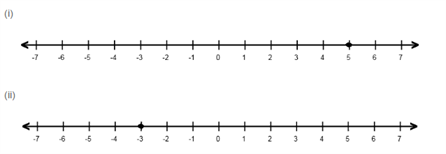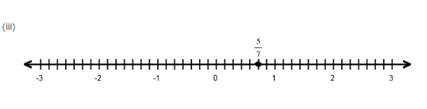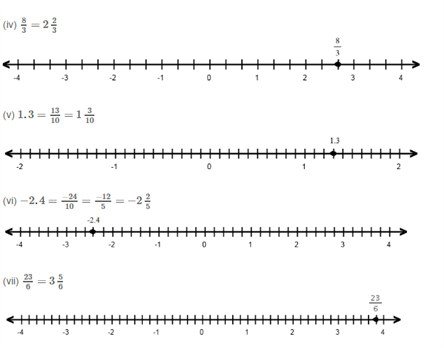Question 2:

Represent each of the following rational numbers on the number line:

I – 5

II — 3

III — 57$\frac{5}{7}$

IV– 83$\frac{8}{3}$

V – 1.3

VI — -24

VII — 236$\frac{23}{6}$

SOLUTION :

(i) Let

X = 14$\frac{1}{4}$ and y = 13$\frac{1}{3}$

Rational number lying between x and y:

12$\frac{1}{2}$ ( x + y) = 12(14+13)$\frac{1}{2}(\frac{1}{4}+\frac{1}{3})$

= 724$\frac{7}{24}$

(ii) Let:

X = 38$\frac{3}{8}$ and y = 25$\frac{2}{5}$

Rational number lying between x and y:

12$\frac{1}{2}$ ( x + y) = 12(38+25)$\frac{1}{2}(\frac{3}{8}+\frac{2}{5})$

= 12(15+1640)$\frac{1}{2}(\frac{15+16}{40})$ = 3180$\frac{31}{80}$

(iii) Let:

X = 1.3 and y = 1.4

Rational number lying between x and y:

12$\frac{1}{2}$ (x + y) = 12$\frac{1}{2}$ (1.3 + 1.4)

= 12$\frac{1}{2}$ (2.7) = 1.35

(iv) Let:

X = 0.75 and y = 1.2

Rational number lying between x and y;

12$\frac{1}{2}$ ( x + y ) = 12$\frac{1}{2}$ ( 0.75 + 1.2)

= 12$\frac{1}{2}$ (1.95) = 0.975

(v) Let:

X= -1 and y = 12$\frac{1}{2}$

Rational number lying between x and y:

12$\frac{1}{2}$ ( x + y ) = 12$\frac{1}{2}$ (1+12)$(-1 + \frac{1}{2})$

= 14$-\frac{1}{4}$

(vi) Let:

X =  34$-\frac{3}{4}$ and y =  25$-\frac{2}{5}$

Rational number lying between x and y:

12$\frac{1}{2}$ ( x + y ) = 12$\frac{1}{2}$ (3425)$(-\frac{3}{4}-\frac{2}{5})$

= 12(15820)=2340$\frac{1}{2}(\frac{-15-8}{20})= -\frac{23}{40}$

Question 3:

Find three rational numbers lying between 15$\frac{1}{5}$  and 14$\frac{1}{4}$

Solution:

Let:

X = 15$\frac{1}{5}$ , Y = 14$\frac{1}{4}$ and n = 3

We know:

d=yxn+1=14153+1=1204=180$d= \frac{y-x}{n+1}=\frac{\frac{1}{4}-\frac{1}{5}}{3+1} = \frac{\frac{1}{20}}{4}=\frac{1}{80}$

So, three rational numbers lying between x and y are:

(x + d), (x + 2d) and ( x + 3d)

= (15+180)$(\frac{1}{5}+\frac{1}{80})$ , (15+280)$(\frac{1}{5}+\frac{2}{80})$ and (15+380)$(\frac{1}{5}+\frac{3}{80})$

= 1780$\frac{17}{80}$ , 1880$\frac{18}{80}$ and 1980$\frac{19}{80}$

Question 4:

Find five rational numbers lying between 25$\frac{2}{5}$ and  34$\frac{3}{4}$

Solution:

Let:

X= 25, y = 34 and n= 5

We know:

d=yxn+1=34255+1=7206=7120$d=\frac{y-x}{n+1} = \frac{\frac{3}{4}-\frac{2}{5}}{5+1} = \frac{\frac{7}{20}}{6}=\frac{7}{120}$

So, five rational numbers between x and y are:

(x + d) ,(x + 2d), (x + 3d), (x + 4d) and (x + 5d)

= (25+7120)(25+14120)(25+21120)(25+28120)and(25+35120)$(\frac{2}{5}+\frac{7}{120})(\frac{2}{5}+\frac{14}{120})(\frac{2}{5}+\frac{21}{120})(\frac{2}{5}+\frac{28}{120}) and (\frac{2}{5}+\frac{35}{120})$

= 55120$\frac{55}{120}$ , 62120$\frac{62}{120}$ , 69120$\frac{69}{120}$ , 76120$\frac{76}{120}$ and 83120$\frac{83}{120}$

Question 5:

Insert 6 rational numbers between 3 and 4.

Solution:

Let:

X= 3, y =4 and n=6

We know:

d = yxn+1$\frac{y-x}{n+1}$ = 436+1$\frac{4-3}{6+1}$ = 17$\frac{1}{7}$

So, six rational numbers between x and y are:

(x + d) ,(x + 2d), (x + 3d), (x + 4d), (x + 5d) and (x + 6d)

= (3+17)$(3+\frac{1}{7})$, (3+27)$(3+\frac{2}{7})$, (3+37)$(3+\frac{3}{7})$ , (3+47)$(3+\frac{4}{7})$, (3+57)$(3+\frac{5}{7})$, (3+67)$(3+\frac{6}{7})$

= 227$\frac{22}{7}$, 237$\frac{23}{7}$, 247$\frac{24}{7}$, 257$\frac{25}{7}$, 267$\frac{26}{7}$ and 277$\frac{27}{7}$

Question 6: Insert 16 rational numbers between 2.1 and 2.2 .

Solution:

Let x = 2.1, y = 2.2 and n= 16

We know,

d = yxn+1$\frac{y-x}{n+1}$ =2.22.116+1$\frac{2.2-2.1}{16+1}$= 0.117$\frac{0.1}{17}$ = 1170$\frac{1}{170}$ = 0.005 (approx)

So, 16 rational numbers between 2.1 and 2.2 are:

(x + d), (x + 2d), . . . . . (x + 16d)

= [ 2.1 + 0.005], [ 2.1 +2( 0.005)]. . . . . . . . . [2.1 + 16(0.005)]

= 2.105, 2.11, 2.115, 2.12, 2.125 , 2.13, 2.135, 2.14, 2.145, 2.15, 2.155, 2.16, 2.165, 2.17, 2.175 and 2.18

Question 7: Without actual division find which of the following rational are terminating decimals

1. 1380$\frac{13}{80}$
2. 724$\frac{7}{24}$
3. 512$\frac{5}{12}$
4. 835$\frac{8}{35}$
5. 16125$\frac{16}{125}$

Solution:

(i) Denominator of 1380$\frac{13}{80}$ is 80.

And,

80 = 24 x 5

Therefore, 80 has no other factors than 2 and 5.

Thus, 1380$\frac{13}{80}$ is a terminating decimal.

(ii) Denominator of 724$\frac{7}{24}$ is 24

And 24 = 23x 3

So, 24 has a prime factor 3, which is other than 2 and 5.

Thus, 724$\frac{7}{24}$ is not a terminating decimal.

(iii) Denominator of 512$\frac{5}{12}$ is 12.

And,

12 = 22x 3

So, 12 has a prime factor 3, which is other than 2 and 5.

Thus, 512$\frac{5}{12}$ is not a terminating decimal.

(iv) Denominator of 835$\frac{8}{35}$ is 35

And,

35 = 7 x 5

So, 35 has a prime factor 7, which is other than 2 and 5.

Thus, 835$\frac{8}{35}$ is not a terminating decimal.

(v) Denominator of 16125$\frac{16}{125}$ is 125.

And,

125 = 53

Therefore, 125 has no other factors than 2 and 5.

Thus, 16125$\frac{16}{125}$ is a terminating decimal.

Question 8: Convert each of the following into a decimal.

1. 58$\frac{5}{8}$
2. 916$\frac{9}{16}$
3. 725$\frac{7}{25}$
4. 1124$\frac{11}{24}$
5. 2512$2\frac{5}{12}$

Solution:

(i) 58$\frac{5}{8}$ = 0.625

By actual division, we have: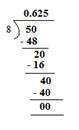(ii) 916$\frac{9}{16}$ = 0.5625

By actual division, we have: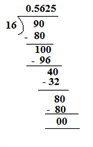(iii) 725$\frac{7}{25}$ = 0.28

By actual division, we have: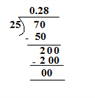(iv) 1124$\frac{11}{24}$ = 0.4583333

By actual division, we have: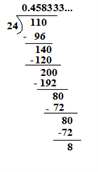(v) 2512$2\frac{5}{12}$ = 2912$\frac{29}{12}$ = 2.416666

By actual division, we have: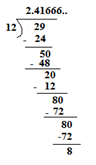Question 9: Express each of the following as a fraction in simplest form.

1. 0. 3¯¯¯$\overline{3}$
2. 1. 3¯¯¯$\overline{3}$
3. 0. 34¯¯¯¯¯$\overline{34}$
4. 3. 14¯¯¯¯¯$\overline{14}$
5. 0. 324¯¯¯¯¯¯¯¯$\overline{324}$
6. 0. 17¯¯¯$\overline{7}$
7. 0.54¯¯¯$\overline{4}$
8. 0.163¯¯¯¯¯$\overline{63}$

Solution:

(i) 0. 3¯¯¯$\overline{3}$

Let x = 0. 3¯¯¯$\overline{3}$

Therefore, X = 0.3333 – – – – – – – – – – – – – – – (1)

10x = 3.3333 – – – – – – – – – – – – – – (2)

On subtracting (1) from (2) we get:

9x = 3

= x = 13$\frac{1}{3}$

Therefore, 0. 3¯¯¯$\overline{3}$ = 13$\frac{1}{3}$

(ii) 1. 3¯¯¯$\overline{3}$

Let x = 1. 3¯¯¯$\overline{3}$

Therefore, X = 1.33333 – – – – – – – – – – – – – (i)

10x = 13.33333 – – – – – – – – – – – (ii)

On subtracting (i) and (ii) we get:

9x = 12

= x = 43$\frac{4}{3}$

Therefore, 1. 3¯¯¯$\overline{3}$ = 43$\frac{4}{3}$

(iii) 0. 34¯¯¯¯¯$\overline{34}$

Let x = 0. 34¯¯¯¯¯$\overline{34}$

Therefore, X = 0.3434… – – – – – – – – – – – – (1)

100x = 34.343434… – – – – – – – – – – (2)

On Subtracting (1) and (2) we get:

99x = 34

= x = 3499$\frac{34}{99}$

Therefore, 0. 34¯¯¯¯¯$\overline{34}$ = 3499$\frac{34}{99}$

(iv) 3. 14¯¯¯¯¯$\overline{14}$

Let x = 3. 34¯¯¯¯¯$\overline{34}$

Therefore, X = 3.1414.. – – – – – – – – – – – (1)

100x = 314.1414… – – – – – – – – – – (2)

On subtracting (1) from (2), we get:

99x = 311

= x = 31199$\frac{311}{99}$

Therefore, 3. 34¯¯¯¯¯$\overline{34}$ = 31199$\frac{311}{99}$

(v) 0. 324¯¯¯¯¯¯¯¯$\overline{324}$

Let x = 0. 324¯¯¯¯¯¯¯¯$\overline{324}$

Therefore, X = 0.324324… – – – – – – – – – – – – – – – (1)

1000x = 324.324324.. – – – – – – – – – – – – (2)

On subtracting (1) from (2), we get:

999x = 324

= x = 324999$\frac{324}{999}$ = 1237$\frac{12}{37}$

Therefore, 0. 324¯¯¯¯¯¯¯¯$\overline{324}$ = 1237$\frac{12}{37}$

(vi) 0. 17¯¯¯$\overline{7}$

Let x = 0.1 7¯¯¯$\overline{7}$

Therefore, X = 0.1777.. – – – – – – – – – – – – – (1)

10x = 1.7777.. – – – – – – – – – – – – – (2)

On subtracting (1) from (2) we get:

90x = 16

= x = 845$\frac{8}{45}$

Therefore, 0.17¯¯¯$\overline{7}$ = 845$\frac{8}{45}$

(vii) 0.54¯¯¯$\overline{4}$

Let x = 0.54¯¯¯$\overline{4}$

Therefore, X = 0.54444…

10x = 5.4444.. – – – – – – – – – – – – – – – (1)

100x = 54.4444… – – – – – – – – – – – – – – (2)

On subtracting (1) from (2) we get:

90x = 49

= x = 4990$\frac{49}{90}$

Therefore, 0.54¯¯¯$\overline{4}$ = 4990$\frac{49}{90}$

(viii) 0.163¯¯¯¯¯$\overline{63}$

Let x = 0.163¯¯¯¯¯$\overline{63}$

Therefore, X = 0.1636363…

10x = 1.6363.. – – – – – – – – – – – – – – – (1)

1000x = 163.6363… – – – – – – – – – – – – – – (2)

On subtracting (1) from (2) we get:

990x = 162

= x = 162990$\frac{162}{990}$= 955$\frac{9}{55}$

Therefore, 0.163¯¯¯¯¯$\overline{63}$ = 955$\frac{9}{55}$

Question 10: Write, whether the given statement is true or false. Give reason.

1. Every natural number is a whole number.
2. Every whole number is a natural number.
3. Every integer is a rational numbers.
4. Every rational number is a whole number.
5. Every terminating decimal is a rational number.
6. Every repeating decimal is a rational number.
7. 0 is a rational number

Solution:

(i) True

Natural numbers start from 1 to infinity and whole numbers start from 0 to infinity; hence, every natural number is a whole number.

(ii) False

0 is a whole number not a natural number, so every whole number is not a natural number.

(iii) True

Every integer can be expressed in the pq$\frac{p}{q}$ form.

(iv) False

Because whole numbers consist only of number of the form p1$\frac{p}{1}$ , where p is a positive number. On the other hand, rational numbers are the numbers whose denominator can be anything except 0.

(v) True

Every terminating decimal can be easily expressed in the pq$\frac{p}{q}$ form.

(vi) True

Every terminating decimal can be easily expressed in the pq$\frac{p}{q}$ form.

(vii) True

0 can be expressed in the form pq$\frac{p}{q}$, so it is a rational number.

### RS Aggarwal Class 9 Solutions Chapter 1 – Real Numbers

RS Aggarwal Solutions of Class 9 prove to be of great value for students looking to score good marks in the exam. The solutions are presented in an easy way, which makes it easier for the students to refer to it while solving the exercise questions. By referring to these RS Aggarwal Class 9 solutions you can clear your doubts and give your preparation the extra edge that it is supposed to require in Class 9th.